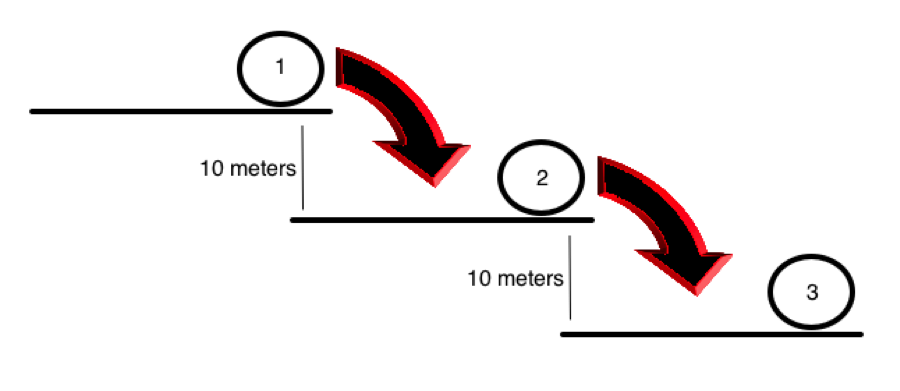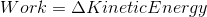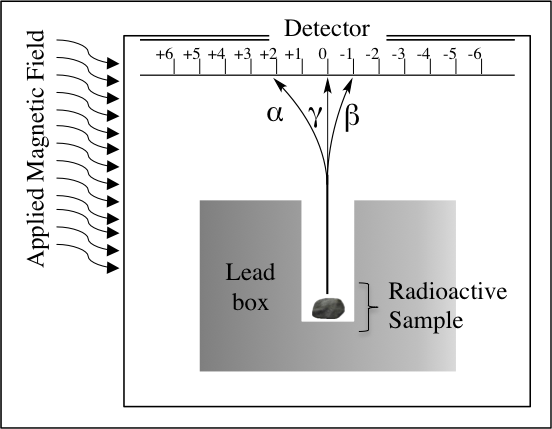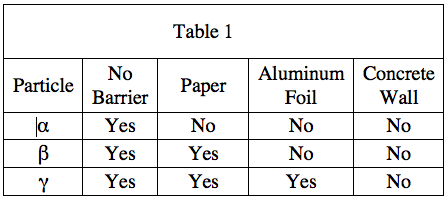## Example Questions

1 2 3 4 5 7 Next →

### Example Question #131 : Physics

A scientist is exploring the nature of energy and work in two experimental systems.

Experiment 1

She first sets up a system with a ball on an inclined plane, and calculates the potential and kinetic energies of the ball at three positions. She places the ball at position 1, stops the ball after rolling to position 2, and then again allows it to roll to position 3. The measured kinetic and potential energies are shown in the table, along with measurements of the force of friction acting on the ball.

 Ball Position Potential Energy Kinetic Energy Force of Friction 1 150 kJ X kJ 0 N 2 75 kJ 75 kJ 0 N 3 X kJ 150 kJ 0 N

Experiment 2

The same scientist then sets up another experiment with the same ball, again at three positions. The ball is provided a slight push, and then allowed to roll down three levels without any additional external input of energy.

She uses the following formulae to calculate the energy levels of the ball:

Potential Energy = Mass of the Ball x Acceleration Due to Gravity x Height of the Ball

Kinetic Energy = (1/2) x Mass of the Ball x (Velocity of the Ball)2

She also measures the internal energy of the ball, a value that she defines as the amount of energy contained in the motion of the molecules that make up the matter of the ball.Position Potential Energy Velocity Internal Energy 1 A 1 m/s 5 kJ 2 B 5.47 m/s 6 kJ 3 C 10.94 m/s 7 kJ

In Experiment 1, if the vertical distance drop is the same from points 2 to 3 as it is from points 1 to 2, which of the following most nearly approximates the value of X?

0 kJ

25 kJ

38 kJ

50 kJ

38 kJ

Explanation:

The magnitude of the height drop is the same as the previous drop, which halved the potential energy. According to the formula in Experiment 2, we should again halve the potential energy, yielding a new potential energy value of 37.5 kJ, or approximately 38 kJ.

### Example Question #1251 : Act Science

A scientist is exploring the nature of energy and work in two experimental systems.

Experiment 1

She first sets up a system with a ball on an inclined plane, and calculates the potential and kinetic energies of the ball at three positions. She places the ball at position 1, stops the ball after rolling to position 2, and then again allows it to roll to position 3. The measured kinetic and potential energies are shown in the table, along with measurements of the force of friction acting on the ball.

 Ball Position Potential Energy Kinetic Energy Force of Friction 1 150 kJ X kJ 0 N 2 75 kJ 75 kJ 0 N 3 X kJ 150 kJ 0 N

Experiment 2

The same scientist then sets up another experiment with the same ball, again at three positions. The ball is provided a slight push, and then allowed to roll down three levels without any additional external input of energy.

She uses the following formulae to calculate the energy levels of the ball:

Potential Energy = Mass of the Ball x Acceleration Due to Gravity x Height of the Ball

Kinetic Energy = (1/2) x Mass of the Ball x (Velocity of the Ball)2

She also measures the internal energy of the ball, a value that she defines as the amount of energy contained in the motion of the molecules that make up the matter of the ball.Position Potential Energy Velocity Internal Energy 1 A 1 m/s 5 kJ 2 B 5.47 m/s 6 kJ 3 C 10.94 m/s 7 kJ

The Law of Conservation of Energy states that energy in the universe cannot be created or destroyed. Which of the following is most likely true in Experiment 2 as the ball moves from position 1 to position 3?

All the kinetic energy lost from position 1 appears as kinetic energy at position 3.

All the kinetic energy lost from position 1 appears as potential energy at position 3.

All the potential energy lost from position 1 appears as kinetic energy at position 3.

Except for 2 kJ, all of the potential energy lost from position 1 appears as kinetic energy at position 3.

Except for 2 kJ, all of the potential energy lost from position 1 appears as kinetic energy at position 3.

Explanation:

The Law of Conervation of Energy states that we cannot create or destroy energy, only that it can change form. Thus, the kinetic energy that appears as increased velocity of the ball at position 3 must come from the potential energy of the ball at position 1. However, since the ball saw an increase in internal energy as well, this 2 kJ increase must be deducted from the final increase in kinetic energy at position 3.

### Example Question #1252 : Act Science

A scientist is exploring the nature of energy and work in two experimental systems.

Experiment 1

She first sets up a system with a ball on an inclined plane, and calculates the potential and kinetic energies of the ball at three positions. She places the ball at position 1, stops the ball after rolling to position 2, and then again allows it to roll to position 3. The measured kinetic and potential energies are shown in the table, along with measurements of the force of friction acting on the ball.

 Ball Position Potential Energy Kinetic Energy Force of Friction 1 150 kJ X kJ 0 N 2 75 kJ 75 kJ 0 N 3 X kJ 150 kJ 0 N

Experiment 2

The same scientist then sets up another experiment with the same ball, again at three positions. The ball is provided a slight push, and then allowed to roll down three levels without any additional external input of energy.

She uses the following formulae to calculate the energy levels of the ball:

Potential Energy = Mass of the Ball x Acceleration Due to Gravity x Height of the Ball

Kinetic Energy = (1/2) x Mass of the Ball x (Velocity of the Ball)2

She also measures the internal energy of the ball, a value that she defines as the amount of energy contained in the motion of the molecules that make up the matter of the ball.Position Potential Energy Velocity Internal Energy 1 A 1 m/s 5 kJ 2 B 5.47 m/s 6 kJ 3 C 10.94 m/s 7 kJ

The work-energy theorem states that the work done on an object is equal to that object's change in kinetic energy:As the ball rolls from position 1 to position 2 in Experiment 1, which of the following is most likely true?

The work done on the ball is positive.

The work done on the ball is 0.

The work done on the ball is negative.

The work done on the ball is 1.

The work done on the ball is positive.

Explanation:

The passage states that the ball has a change in kinetic energy that is positive as it rolls down the ramp. Based on the equation in the question, this means that the work done on the ball must also be positive.

### Example Question #134 : Physics

A scientist is exploring the nature of energy and work in two experimental systems.

Experiment 1

She first sets up a system with a ball on an inclined plane, and calculates the potential and kinetic energies of the ball at three positions. She places the ball at position 1, stops the ball after rolling to position 2, and then again allows it to roll to position 3. The measured kinetic and potential energies are shown in the table, along with measurements of the force of friction acting on the ball.

 Ball Position Potential Energy Kinetic Energy Force of Friction 1 150 kJ X kJ 0 N 2 75 kJ 75 kJ 0 N 3 X kJ 150 kJ 0 N

Experiment 2

The same scientist then sets up another experiment with the same ball, again at three positions. The ball is provided a slight push, and then allowed to roll down three levels without any additional external input of energy.

She uses the following formulae to calculate the energy levels of the ball:

Potential Energy = Mass of the Ball x Acceleration Due to Gravity x Height of the Ball

Kinetic Energy = (1/2) x Mass of the Ball x (Velocity of the Ball)2

She also measures the internal energy of the ball, a value that she defines as the amount of energy contained in the motion of the molecules that make up the matter of the ball.Position Potential Energy Velocity Internal Energy 1 A 1 m/s 5 kJ 2 B 5.47 m/s 6 kJ 3 C 10.94 m/s 7 kJ

As the velocity of an object is increased fourfold, its kinetic energy most likely increases by a factor of:

4

16

8

2

16

Explanation:

The formula in the passage states that the increase in velocity is squared to find in the change in kinetic energy. Thus, if you quadruple the velocity, you increase the overal kinetic energy by a factor of 16.

### Example Question #1251 : Act Science

A scientist is exploring the nature of energy and work in two experimental systems.

Experiment 1

She first sets up a system with a ball on an inclined plane, and calculates the potential and kinetic energies of the ball at three positions. She places the ball at position 1, stops the ball after rolling to position 2, and then again allows it to roll to position 3. The measured kinetic and potential energies are shown in the associated table, along with measurements of the force of friction acting on the ball.

 Ball Position Potential Energy Kinetic Energy Force of Friction 1 150 kJ X kJ 0 N 2 75 kJ 75 kJ 0 N 3 X kJ 150 kJ 0 N

Experiment 2

The same scientist then sets up another experiment with the same ball, again at three positions. The ball is provided a slight push, and then allowed to roll down three levels without any additional external input of energy.

She uses the following formulae to calculate the energy levels of the ball:

Potential Energy = Mass of the Ball x Acceleration Due to Gravity x Height of the Ball

Kinetic Energy = (1/2) x Mass of the Ball x (Velocity of the Ball)2

She also measures the internal energy of the ball, a value that she defines as the amount of energy contained in the motion of the molecules that make up the matter of the ball.Position Potential Energy Velocity Internal Energy 1 A 1 m/s 5 kJ 2 B 5.47 m/s 6 kJ 3 C 10.94 m/s 7 kJ

As the height of an object is increased fourfold, its potential energy most likely increases by a factor of:

32

2

16

4

4

Explanation:

Unlike kinetic energy, the formula for potential energy in the passage indicates that the potential energy is only directly proportional to the height of the object, not its square. Thus, the potential energy increases by the same factor as the height increases.

### Example Question #136 : Physics

Experiment 1

A scientist develops the following setup, shown in Figure 1 below, to study the charges of radioactive particles. A radioactive sample is placed into a lead box that has an open column such that the particles can only exit from one direction. A detector is placed in front of the opening. A metric ruler (centimeters (cm)), is aligned on the detector such that zero is directly in front of the opening of the column, with the positive values extending to the left and the negative values to the right. On the left side of the experimental setup, there is a device that generates a magnetic field that attracts positively charged particles and repels negatively charged particles.Figure 1.

The device detects particles in three different places: alpha, α; beta, β; and gamma, γ; as labeled in Figure 1. The paths these particles take from the source of radioactivity are shown.

Experiment 2

A different scientist finds the following data, shown in Table 1, about the energies of the α, β, and γ particles by observing what kinds of materials through which the particles can pass. This scientist assumes that the ability of particles to pass through thicker and denser barriers is indicative of higher energy. Table 1 summarizes whether or not each type of particle was detected when each of the following barriers is placed between the radioactivity source and the detector. The paper and aluminum foil are both 1 millimeters thick, and the concrete wall is 1 meter thick.It is discovered that a certain element in the Earth's crust emits beta particles, and furthermore, that beta particles can cause cancer. Should architects building houses in the areas where this phenomenon is found in high abundance be mandated to build basements with concrete walls with a minimum thickness of one meter?

Yes, because beta particles are only stopped by concrete walls at least one meter thick.

No, because beta particles are not energetic enough to pass through an aluminum foil sheet.

No, because beta particles are negatively charged, and thus will bounce off the basement's external walls.

Yes, because there will be very large numbers of beta particles emitted from the Earth's crust in these areas.

No, because beta particles are not energetic enough to pass through an aluminum foil sheet.

Explanation:

Table 1 shows that a simple sheet of aluminum foil can deflect beta particles. Thus, we know that any basement walls will be sufficient to deflect beta particles, and extra measures to build thick walls would be a waste of materials.

### Example Question #137 : Physics

Experiment 1

A scientist develops the following setup, shown in Figure 1 below, to study the charges of radioactive particles. A radioactive sample is placed into a lead box that has an open column such that the particles can only exit from one direction. A detector is placed in front of the opening. A metric ruler (centimeters (cm)), is aligned on the detector such that zero is directly in front of the opening of the column, with the positive values extending to the left and the negative values to the right. On the left side of the experimental setup, there is a device that generates a magnetic field that attracts positively charged particles and repels negatively charged particles.Figure 1.

The device detects particles in three different places: alpha, α; beta, β; and gamma, γ; as labeled in Figure 1. The paths these particles take from the source of radioactivity are shown.

Experiment 2

A different scientist finds the following data, shown in Table 1, about the energies of the α, β, and γ particles by observing what kinds of materials through which the particles can pass. This scientist assumes that the ability of particles to pass through thicker and denser barriers is indicative of higher energy. Table 1 summarizes whether or not each type of particle was detected when each of the following barriers is placed between the radioactivity source and the detector. The paper and aluminum foil are both 1 millimeters thick, and the concrete wall is 1 meter thick.Based on Experiment 2, the scientist could conclude that the particles, in order of increasing energy, are __________.

γ < α < β

α < γ < β

α < β < γ

β < α < γ

α < β < γ

Explanation:

In the description of Experiment 2, it is stated that it assumed that the thicker and denser the materials a particle can pass through, the higher the energy of the particle. Thus, since the alpha particle can be stopped even by a piece of paper, while the other two particles can pass through, it must have the lowest energy of the three particles measured. Secondly, the beta particle can be stopped by aluminum foil, while gamma particles pass through it, so the beta particle is second lowest in energy. Lastly, the gamma particles may only be stopped by a one-meter-thick concrete wall, so they are the highest in energy.

### Example Question #138 : Physics

Experiment 1

A scientist develops the following setup, shown in Figure 1 below, to study the charges of radioactive particles. A radioactive sample is placed into a lead box that has an open column such that the particles can only exit from one direction. A detector is placed in front of the opening. A metric ruler (centimeters (cm)), is aligned on the detector such that zero is directly in front of the opening of the column, with the positive values extending to the left and the negative values to the right. On the left side of the experimental setup, there is a device that generates a magnetic field that attracts positively charged particles and repels negatively charged particles.Figure 1.

The device detects particles in three different places: alpha, α; beta, β; and gamma, γ; as labeled in Figure 1. The paths these particles take from the source of radioactivity are shown.

Experiment 2

A different scientist finds the following data, shown in Table 1, about the energies of the α, β, and γ particles by observing what kinds of materials through which the particles can pass. This scientist assumes that the ability of particles to pass through thicker and denser barriers is indicative of higher energy. Table 1 summarizes whether or not each type of particle was detected when each of the following barriers is placed between the radioactivity source and the detector. The paper and aluminum foil are both 1 millimeters thick, and the concrete wall is 1 meter thick.A new type of radioactive material is discovered. A sample of it is placed into the lead chamber of the experimental setup described in Experiment 1. The detector detects radioactive particles at +1 cm. Which one of the following statements is true?

The material emits α particles.

The material emits β particles.

None of these

The material emits γ particles.